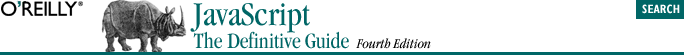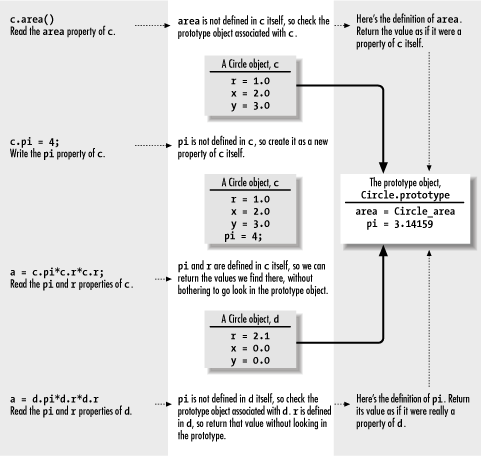home | O'Reilly's CD bookshelfs | FreeBSD | Linux | Cisco | Cisco Exam8.4. Prototypes and Inheritance

JavaScript objects "inherit" properties from a prototype object. Every object has a prototype; all of the properties of the prototype object appear to be properties of any objects for which it is a prototype. That is, each object inherits properties from its prototype.

Prototypes were introduced in JavaScript 1.1; they are not supported in the now obsolete JavaScript 1.0.

Note that inheritance occurs automatically, as part of the process of looking up a property value. Properties are not copied from the prototype object into new objects; they merely appear as if they were properties of those objects. This has two important implications. First, the use of prototype objects can dramatically decrease the amount of memory required by each object, since the object can inherit many of its properties. The second implication is that an object inherits properties even if they are added to its prototype after the object is created.

Each class has one prototype object, with one set of properties. But there are potentially many instances of a class, each of which inherits those prototype properties. Because one prototype property can be inherited by many objects, JavaScript must enforce a fundamental asymmetry between reading and writing property values. When you read property p of an object o, JavaScript first checks to see if o has a property named p. If it does not, it next checks to see if the prototype object of o has a property named p. This is what makes prototype-based inheritance work.

When you write the value of a property, on the other hand, JavaScript does not use the prototype object. To see why, consider what would happen if it did: suppose you try to set the value of the property o.p when the object o does not have a property named p. Further suppose that JavaScript goes ahead and looks up the property p in the prototype object of o and allows you to set the property of the prototype. Now you have changed the value of p for a whole class of objects -- not at all what you intended.Figure 8-1. Objects and prototypes

Let's move from an abstract discussion of prototype inheritance to a concrete example. Suppose we define a Circle( ) constructor function to create objects that represent circles. The prototype object for this class is Circle.prototype, so we can define a constant available to all Circle objects like this:

The prototype object of a constructor is created automatically by JavaScript. In most versions of JavaScript, every function is automatically given an empty prototype object, just in case it is used as a constructor. In JavaScript 1.1, however, the prototype object is not created until the function is used as a constructor for the first time. This means that if you require compatibility with JavaScript 1.1, you should create at least one object of a class before you use the prototype object to assign methods and constants to objects of that class. So, if we have defined a Circle( ) constructor but have not yet used it to create any Circle objects, we'd define the constant property pi like this:

//First create and discard a dummy object; forces prototype object creation.
new Circle ( );
//Now we can set properties in the prototype.
Circle.prototype.pi = 3.14159'

Example 8-4. Defining a Circle class with a prototype object

// Define a constructor method for our class.
// Use it to initialize properties that will be different for
// each individual Circle object.
function Circle(x, y, r)
{
this.x = x;  // The X-coordinate of the center of the circle
this.y = y;  // The Y-coordinate of the center of the circle
this.r = r;  // The radius of the circle
}

// Create and discard an initial Circle object.
// This forces the prototype object to be created in JavaScript 1.1.
new Circle(0,0,0);

// Define a constant: a property that will be shared by
// all circle objects. Actually, we could just use Math.PI,
// but we do it this way for the sake of instruction.
Circle.prototype.pi = 3.14159;

// Define a method to compute the circumference of the circle.
// First declare a function, then assign it to a prototype property.
// Note the use of the constant defined above.
function Circle_circumference( ) { return 2 * this.pi * this.r; }
Circle.prototype.circumference = Circle_circumference;

// Define another method. This time we use a function literal to define
// the function and assign it to a prototype property all in one step.
Circle.prototype.area = function( ) { return this.pi * this.r * this.r; }

// The Circle class is defined.
// Now we can create an instance and invoke its methods.
var c = new Circle(0.0, 0.0, 1.0);
var a = c.area( );
var p = c.circumference( );